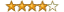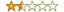# Statistics-Basic reviews

### Statistics-Basic (1.6601)Dox are standard, but assumes you remember all your statistics.
You probably want correlation, not covariance (though the latter is likely to pop into your mind if you're thinking about ANOVA and colinearity). I also ended up getting a reminder from Math::NumberCruncher that, "Correlation ranges from 1 to -1, with a correlation of zero meaning no correlation exists between the two variables."

It's unfortunate that (like M::NC) the co* methods only operate on two vectors. Therefore you may need something like:

use Math::Combinatorics;

use Statistics::Basic qw(:all);

my \$combos = Math::Combinatorics->new(count=>2, data=>[@vectors]);

while(my @combo = \$combos->next_combination){

my \$cor = correlation( @combo );

print "The correlation is \$cor for vectors:\n\$combo\n\$combo\n" if abs(\$cor) > .66;

}

### Statistics-Basic (1.6001)

(I've made code changes and doc changes for all these reviews. Thanks for the feedback you guys.)

### Statistics-Basic (1.6003)This is a nice module, and for simple averages or standard deviations it's significantly faster than Statistics::Descriptive. By default, standard deviation results are population (n), not sample (n-1).

### Statistics-Basic (0.42)What a horrible interface: why call a function to take the mean of your data when, instead, you can simply create a new "mean object" with the data, then "query" it to get the result? </sarcasm> Plus there's not much functionality. If you want statistics, use Statistics::Descriptive.